# Sampling Methods and the Central Limit Theorem Chapter

• Slides: 16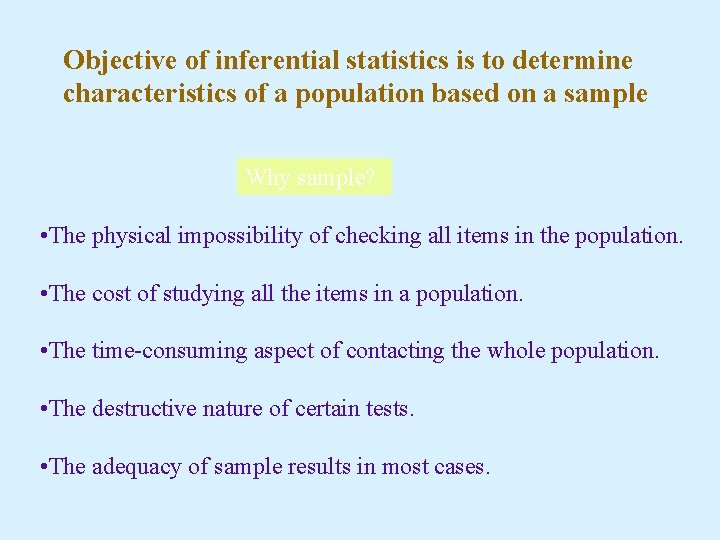Objective of inferential statistics is to determine characteristics of a population based on a sample Why sample? • The physical impossibility of checking all items in the population. • The cost of studying all the items in a population. • The time-consuming aspect of contacting the whole population. • The destructive nature of certain tests. • The adequacy of sample results in most cases.Sampling Methods Simple Random Sample: A sample selected so that each item or person in the population has the same chance of being included. One can also a table of random numbers (Appendix E)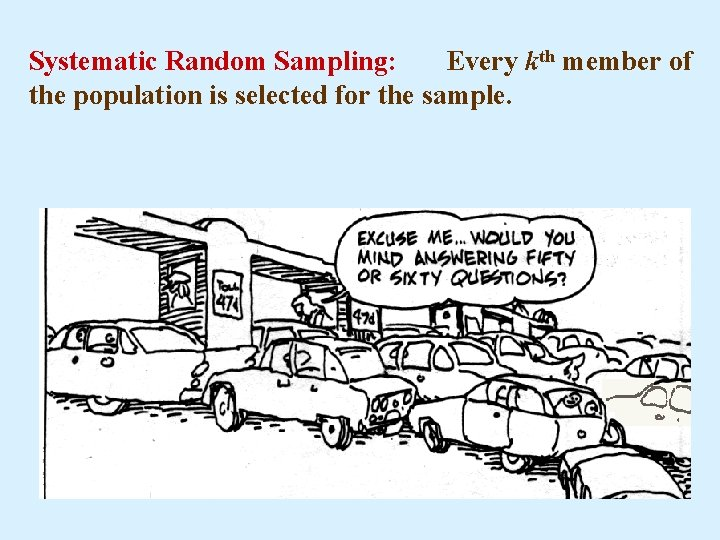Systematic Random Sampling: Every kth member of the population is selected for the sample.Stratified Random Sampling: A population is first divided into subgroups, called strata, and a sample is selected from each stratum. Eg. College students may be stratified into freshmen, sophomore, etc. or simply male and femaleCluster Sampling: A population is first divided into primary units then samples are selected from the primary units.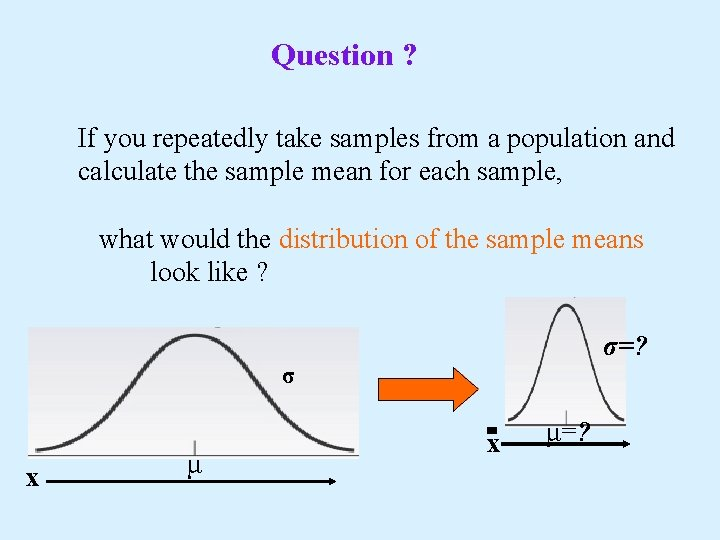Question ? If you repeatedly take samples from a population and calculate the sample mean for each sample, what would the distribution of the sample means look like ? σ=? σ x μ=?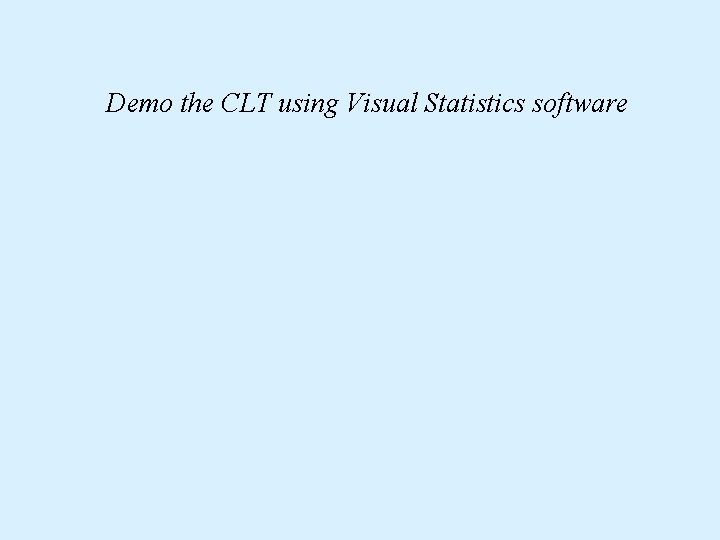Demo the CLT using Visual Statistics software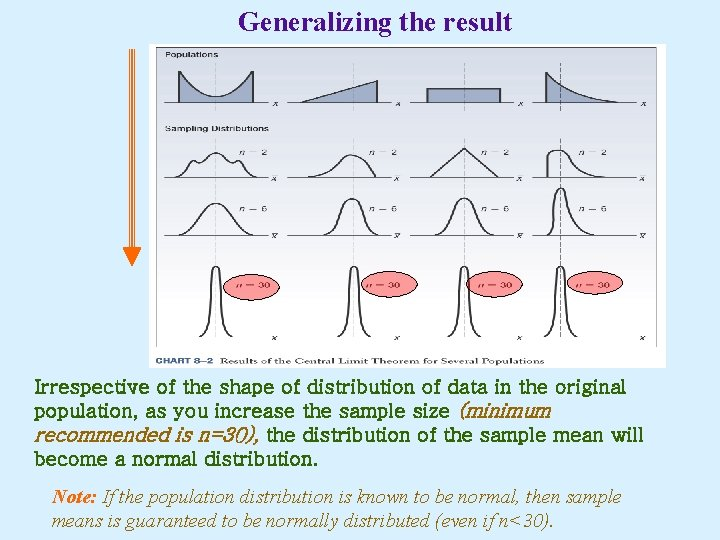Generalizing the result Irrespective of the shape of distribution of data in the original population, as you increase the sample size (minimum recommended is n=30), the distribution of the sample mean will become a normal distribution. Note: If the population distribution is known to be normal, then sample means is guaranteed to be normally distributed (even if n<30).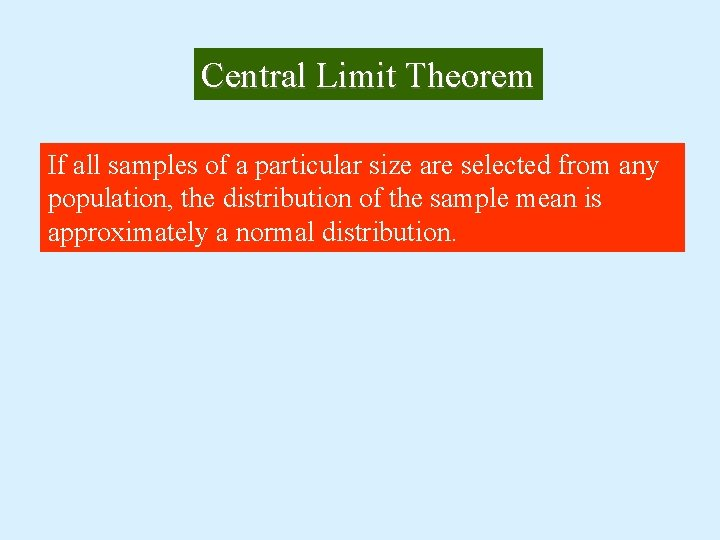Central Limit Theorem If all samples of a particular size are selected from any population, the distribution of the sample mean is approximately a normal distribution.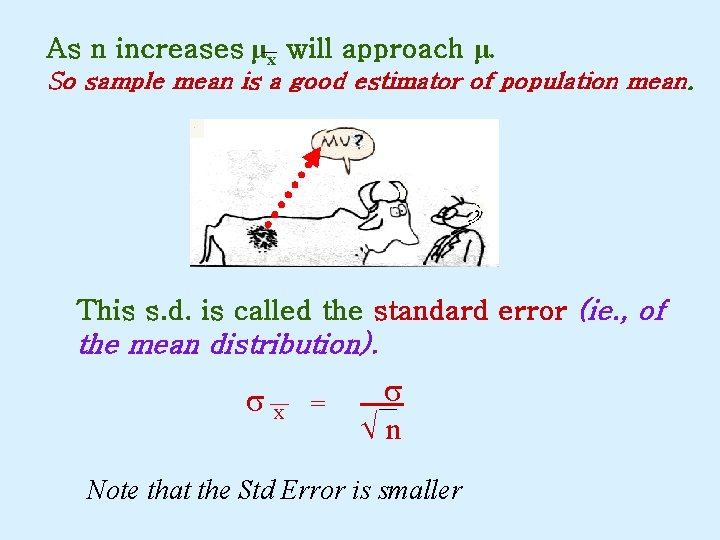As n increases μx will approach μ. So sample mean is a good estimator of population mean. This s. d. is called the standard error (ie. , of the mean distribution). sx = s n Note that the Std Error is smallerVariance of the sample mean distribution x 1 is mean of Var(x) = Var (x 1 + x 2 +…+xn) Where sample 1, x 2 is …) n = 1 [Var(x 1) + Var(x 2) + … +Var(xn)] n 2 = 1 [σ2 + … + σ2] = 1 [n. σ2] = n 2 σx 2 = σ2 n therefore, Standard Deviation = σ/√n (Remember this formula!) n σ2 n 2σ x Distribution of population μ Std. Error σ/√n x Distribution of sample μ The Z score formula for the distribution of sample means is: Compare with Chapter 7 formula: -m X z= σ nPractice! Historically, the average sales per customer at a tire store is known to be \$85, with a s. d. of \$9. You take a random sample of 40 customers. What is the probability the mean expenditure for this sample will be \$87 or more? Z= 87 – 85 = 2 = 1. 41 9/√ 40 1. 42 From Appendix D, prob. for this Z-score is 0. 4207. The prob for sample mean to exceed Z=1. 41 is 0. 5 – 0. 4207. Hence, the answer is 0. 0793.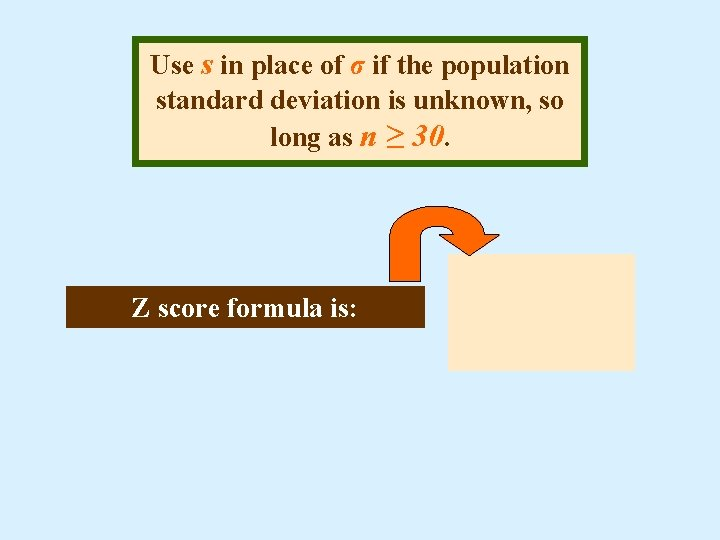Use s in place of σ if the population standard deviation is unknown, so long as n ≥ 30. Z score formula is: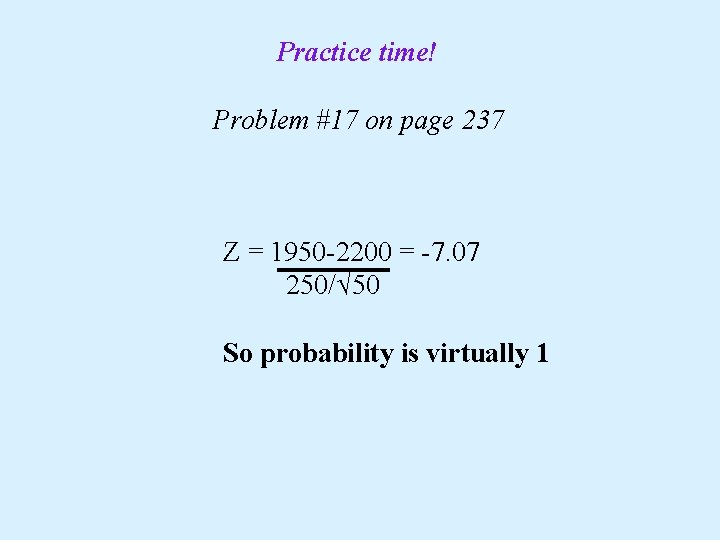Practice time! Problem #17 on page 237 Z = 1950 -2200 = -7. 07 250/√ 50 So probability is virtually 1